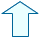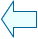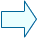STATIONARY POINTS Analysis

A function y=f(x) has a STATIONARY POINT at xo when f'(xo)=0

In this case we know that the tangent to the curve is horizontal at xo .

You have already seen that there can be a local maximum or minimum depending on the sign of f''(xo), but when f''(xo) is also equal to 0, there could be a point of inflexion.

So, what happens when successive derivatives are equal to 0 at xo?

EXAMPLE 1

Look at the graph of the function y=x4-1 in the window and note that it has a minimum at x=0.

• The value of the derivative y'=4x3 is zero at x=0
The value of the second derivative y''=12x2 is also zero at x=0 as is that of the third derivative y'''=24x ; the first derivative not equal to zero being the fourth, which is also positive.

 Change the value of x in the window and check the above. Then, make the value of the FUNCTION equal to 2 and the graph of y=1-x4 will appear. What is its behaviour at x=0? Change the value of x again.

• Note that in both cases the first derivative not to have a value of zero at x=0 is of an EVEN order.

 If the first derivative not to have a value of zero at xo is of an EVEN order, f has either a maximum or a minimum at xo depending on whether it is positive or negative.

Look at the graph of the function y=x5 in the window. As you can see in the graph, f has a point of inflexion at x=0.

• We can see that the value of the derivative y'=5x4 is zero at x=0,
the second derivative y''=20x3 also has a value of zero at x=0 along with the third derivative y'''=60x2 and the fourth; the fifth derivative is the only one different to zero.

 Change the value of x to check this in the window.

• Note that the first derivative not to have a value of zero at x=0 is of an ODD order.

 If the first derivative not to have a value of zero at xo is of an ODD order, f has a point of inflexion at xo.María José García CebrianSpanish Ministry of Education. Year 2001